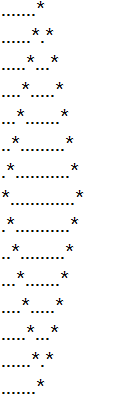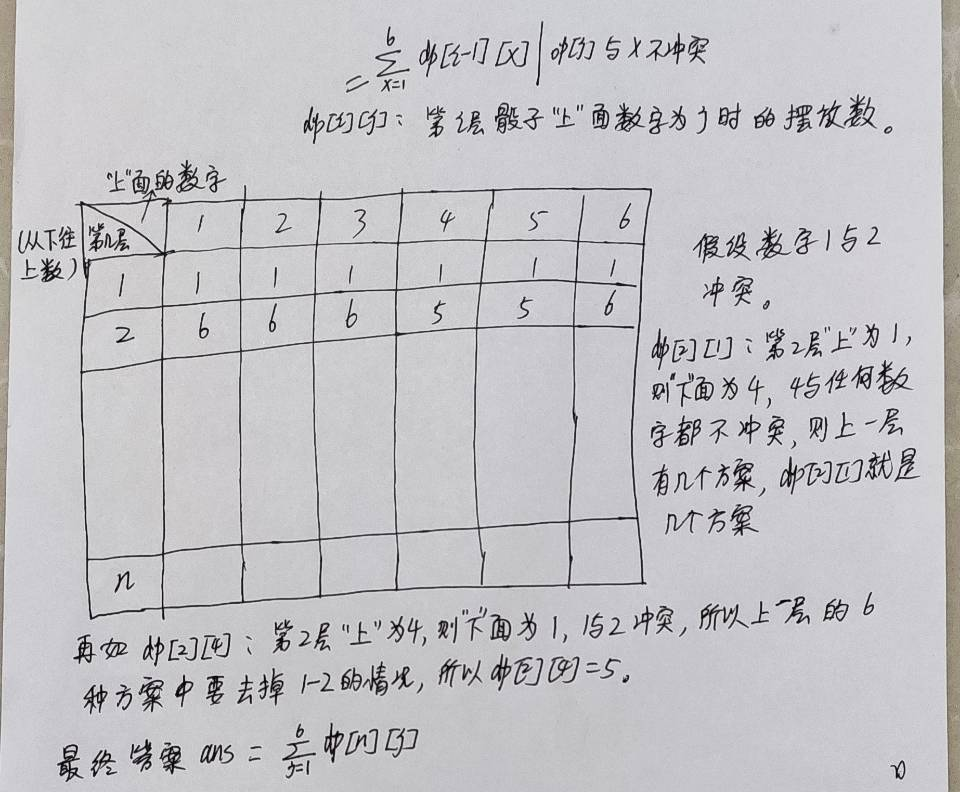## 2015年第六届蓝桥杯JavaA组_码畜也有梦想的博客-程序员信息网

——————————————————————————————————————————————————————

``````public class Main {

public static void main(String[] args) {

int ans = 0;
for(int i = 1543;i > 0;) {

if((i & 1) == 0) {
//偶数
i /= 2;
}else {

ans++;
i = (i - 1) / 2;
}
}
System.out.println(ans);
}
}
``````

``````import java.util.Calendar;

public class Main {

public static void main(String[] args) {

Calendar c = Calendar.getInstance();
c.set(2014, 10, 9);
System.out.println(c.getTime().toLocaleString());
}
}
``````

1~9的数字可以组成3个3位数，设为：A,B,C, 现在要求满足如下关系：
B = 2 * A
C = 3 * A

``````public class Main {

static int[] arr = {
1,2,3,4,5,6,7,8,9};
//全排列
public static void main(String[] args) {

f(0);
}

private static void f(int k) {

if(k == 9) {

int a = arr * 100 + arr * 10 + arr;
int b = arr * 100 + arr * 10 + arr;
int c = arr * 100 + arr * 10 + arr;
if(b == 2 * a && c == 3 * a) System.out.print(a + " ");
}
for(int i = k;i < 9;i++) {

int tmp = arr[i];
arr[i] = arr[k];
arr[k] = tmp;

f(k + 1);

tmp = arr[i];
arr[i] = arr[k];
arr[k] = tmp;
}
}
}
``````

``````public static int f(int n, int m)
{
n = n % m;
Vector v = new Vector();

for(;;)
{
n *= 10;
n = n % m;
if(n==0) return 0;
if(v.indexOf(n)>=0)  ____return v.size() - v.indexOf(n) ___ ;  //填空
}
}
``````

——————————————————————————————————————————————————``````public class A
{
public static void f(int n)
{
String s = "*";
for(int i=0; i<2*n-3; i++) s += ".";
s += "*";

String s1 = s + "\n";
String s2 = "";

for(int i=0; i<n-1; i++){
//System.out.println("=>"+s);
s = "." + ____s.substring(0, s.length() - 3)______ + "*";  //填空
s1 = s + "\n" + s1;
s2 += s + "\n";
}
System.out.println(s1+s2);
}

public static void main(String[] args)
{
f(8);
}
}
``````

————————————————————————————————————————————————————

1+2+3+…+10 * 11+12+…+27 * 28+29+…+49 = 2015

——————————————————————————————————————————————————————

``````public class Main {

public static void main(String[] args) {

//枚举两个 * 的位置
for(int i = 1;i <= 46;i++) {
//第一个 * 左边的数字
for(int j = i + 2;j <= 48;j++) {
//第二个 * 左边的数字
//只比较变动的两处产生的差值
if(i * (i + 1) - (i + (i + 1)) + j * (j + 1) - (j + (j + 1)) == 2015 - 1225)
System.out.println(i + " " + j);
}
}
}
}
``````

——————————————————————————————————————————————————

``````public class Main {

static int ans;//3598180

public static void main(String[] args) {

f(0, 0);
System.out.println(ans);
}
/**
* @param k 目前考虑到点数为 k 的牌型
* @param count 手中已有的牌的总数
*/
private static void f(int k, int count) {

if(count > 13 || k > 13) return ;//剪枝
if(k == 13 && count == 13) {

ans++;
return ;
}
for(int i = 0;i < 5;i++) {
//点数为 k 的牌型，都可能抓 0---4张
f(k + 1, count + i);
}
}
}
``````

X星球居民小区的楼房全是一样的，并且按矩阵样式排列。其楼房的编号为1,2,3…

1 2 3 4 5 6
12 11 10 9 8 7
13 14 15 …

w为排号宽度，m,n为待计算的楼号。

6 8 2

4

4 7 20

5

CPU消耗 < 1000ms

————————————————————————————————————————————————————

``````import java.util.Scanner;

public class Main {

public static void main(String[] args) {

Scanner sc = new Scanner(System.in);
int w = sc.nextInt();
int m = sc.nextInt();
int n = sc.nextInt();
sc.close();

int rm = m % w == 0 ? m / w : m / w + 1; //m 的row
int rn = n % w == 0 ? n / w : n / w + 1; //n 的row
int cm = 0;// m 的col
int cn = 0;// n 的col
if(rm % 2 == 0) {
//偶数行
cm = rm * w - m + 1;//rm * w 是 m 所在行的最大值
}else {

cm = w - (rm * w - m);
}
if(rn % 2 == 0) {
//偶数行
cn = rn * w - n + 1;
}else {

cn = w - (rn * w - n);
}
System.out.println(Math.abs(rm - rn) + Math.abs(cm - cn));
}

}
``````

「输入格式」

n表示骰子数目

「输出格式」

「样例输入」
2 1
1 2

「样例输出」
544

「数据范围」

CPU消耗 < 2000ms

————————————————————————————————————————————————————

``````import java.util.Scanner;

public class Main {

static final int MOD = 1000000007;
static int[] op = new int; //op[i]表示 i 的 对面是 op[i]
static boolean[][] conflict = new boolean;
static int m;
static int n;

public static void main(String[] args) {

//初始化op数组
op = 4;
op = 1;
op = 5;
op = 2;
op = 6;
op = 3;

Scanner sc = new Scanner(System.in);
n = sc.nextInt();
m = sc.nextInt();
for(int i = 0;i < m;i++) {

int x = sc.nextInt();//冲突对
int y = sc.nextInt();
conflict[x][y] = true;
conflict[y][x] = true;
}
sc.close();

long ans = 0;
for(int up = 1; up <= 6;up++) {
//第一层的骰子"上"面的数字，六种可能
ans = (ans + 4 * f(up, n - 1)) % MOD;//每一层都可以转出四种方案
}
System.out.println(ans);
}
/**
* 上一层定好了朝上的数字为up的情况下，垒好count个骰子的方案数
* @param up 上一层骰子"上"面的数字
* @param count
* @return
*/
private static long f(int up, int count) {

//前面 n 个骰子定好了，这是1种方案，但是四周的面可以转动，"前"面可以有4种选择，所以是四种方案
if(count == 0) return 4;
long ans = 0;
for(int upp = 1;upp <= 6;upp++) {

//上一层骰子的"下"面 和 这一层骰子的"上"面冲突的话
if(conflict[op[up]][upp]) continue;
ans = (ans + f(upp, count - 1)) % MOD;
}
return ans;
}
}
````````````import java.util.Scanner;

public class Main {

static final int MOD = 1000000007;
static int[] op = new int; //op[i]表示 i 的 对面是 op[i]
static boolean[][] conflict = new boolean;
static int m;
static int n;

public static void main(String[] args) {

Scanner sc = new Scanner(System.in);
n = sc.nextInt();
m = sc.nextInt();
for(int i = 0;i < m;i++) {

int x = sc.nextInt();//冲突对
int y = sc.nextInt();
conflict[x][y] = true;
conflict[y][x] = true;
}
sc.close();

long[][] dp = new long;//每一层只与上一层有关，所以两层就够了
//初始化dp
for(int j = 1;j <= 6;j++) dp[j] = 1;
int cur = 0;//当前行
//迭代层数
for(int level = 2;level <= n;level++) {

cur = 1 - cur;
//尝试"上"面的数字
for(int j = 1;j <= 6;j++) {

dp[cur][j] = 0;
//将与 op[j]不冲突的上一层格子的里面的数累加起来
for(int i = 1;i <= 6;i++) {

if(conflict[op[j]][i]) continue;//冲突的面朝上是不可取的
dp[cur][j] = (dp[cur][j] + dp[1 - cur][i]) % MOD;
}
}
}
long sum = 0;
//求最终答案，即第 n 层的总和
for(int k = 1;k <= 6;k++) {

sum = (sum + dp[cur][k]) % MOD;
}
//每一层都可旋转处四种方案，所以还要乘以4^n
long ans = 1;
long tmp = 4;
long p = n;
//快速幂求 4^n
while(p != 0) {

if((p & 1) == 1) ans = (ans * tmp) % MOD;
tmp = (tmp * tmp) % MOD;
p >>= 1;
}
System.out.println((ans * sum) % MOD);
}
}
``````

### densenet与resneXt的巧妙结合-DPN网络_春枫琰玉的博客-程序员信息网

DPN是在resneXt,denseNet之后，对resnet系列的进一步创新，作者巧妙的将resnet与denseNet相结合，提出了dual path architectures，构造了DPN网络结构。 那么DPN的创新点有哪些呢？如下：DPN具有更小的模型以及更少的计算量，具有更好的训练速度。作者在论文中的描述为：[In particular，on the ImageNet..._1671465600

### Python 2.7.9 Demo - 获取调用的参数_dongjizheng9270的博客-程序员信息网

#coding=utf-8#!/usr/bin/pythonimport sys;print("The command line parameters are : ");for i in range(0, len(sys.argv)) : print str(i) + ' -&gt; ' + sys.argv[i];日志...

### JAVA 赛码网|笔试时输入输出的控制_张小李的风的博客-程序员信息网

JAVA 笔试输入用例因为习惯了力扣的刷题模式，在刚刚接触互联网笔试的时候，被输入的控制搞得很头痛，尤其是说明有多个测试用例的时候，很容易只控制了单个测试用例，导致本来算法思路没问题，最后无法AC。两道例题，给自己做一个笔记。忽略题解，就输入控制可以参考。题目1import java.util.*;public class Main{public static void main(String[] args) { Scanner cin = new Scanner(System.in);

### 在线教育软件开发 教育APP软件开发分析_juzimvp的博客-程序员信息网

随着互联网的快速发展，教育机构也纷纷改变了发展模式，教育APP软件的开发大受欢迎，司科认为教育软件的开发不仅能让教学资源共享，更能促进教育行业的发展。　　为什么教育类APP软件值得开发　　1、使用教育APP的用户规模大　　开发学习教育APP软件，是因为用户规模非常大，因为无论是全年龄段的人群还是学前儿童，都有通过APP学习的需求。加上不少的学习类教育移动APP功能优化速度是非常快的...

### 宽度优先搜索------迷宫的最短路径_Oanakiaja的博客-程序员信息网

·迷宫的最短路径　　给定一个大小为N×M的迷宫。迷宫由通道和墙壁组成，每一步可以向邻接的上下左右四个的通道移动。请求出从起点到终点所需的最小步数。请注意，本题假定从起点一定可以移动到终点。（N,M≤100）（'#', '.' , 'S', 'G'分别表示墙壁、通道、起点和终点）输入：10 10#S######.#......#..#.#.##.##.#.#...........

### elementUI el-select中el-option中插入图片_Xv威的博客-程序员信息网_option 加图片

el-select中el-option中插入图片先看效果上代码&lt;el-select v-model="form.promotionGroupStyle.id" style="width: 355px;" filterable clearable @change="QueryPromotionGroupStyle" &gt; &lt;el-option v-for="item in promotionGroupStyles" :key="item.id":label="item.name"